CAT  >  IIFT Data Interpretation MCQ - 2

# IIFT Data Interpretation MCQ - 2

Test Description

## 18 Questions MCQ Test IIFT Mock Test Series | IIFT Data Interpretation MCQ - 2

IIFT Data Interpretation MCQ - 2 for CAT 2022 is part of IIFT Mock Test Series preparation. The IIFT Data Interpretation MCQ - 2 questions and answers have been prepared according to the CAT exam syllabus.The IIFT Data Interpretation MCQ - 2 MCQs are made for CAT 2022 Exam. Find important definitions, questions, notes, meanings, examples, exercises, MCQs and online tests for IIFT Data Interpretation MCQ - 2 below.
Solutions of IIFT Data Interpretation MCQ - 2 questions in English are available as part of our IIFT Mock Test Series for CAT & IIFT Data Interpretation MCQ - 2 solutions in Hindi for IIFT Mock Test Series course. Download more important topics, notes, lectures and mock test series for CAT Exam by signing up for free. Attempt IIFT Data Interpretation MCQ - 2 | 18 questions in 20 minutes | Mock test for CAT preparation | Free important questions MCQ to study IIFT Mock Test Series for CAT Exam | Download free PDF with solutions
 1 Crore+ students have signed up on EduRev. Have you?
IIFT Data Interpretation MCQ - 2 - Question 1

### Group Question Answer the following question based on the information given below. Analyze the charts given below that show the energy consumption and energy production of XYZ country from various sources. The difference in production and consumption of energy from any source is bridged through external trade (i.e. export or import) of energy from the same source. All the values are given in Terawatt-hours (Twh).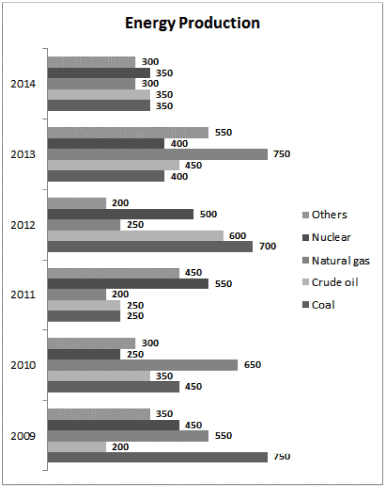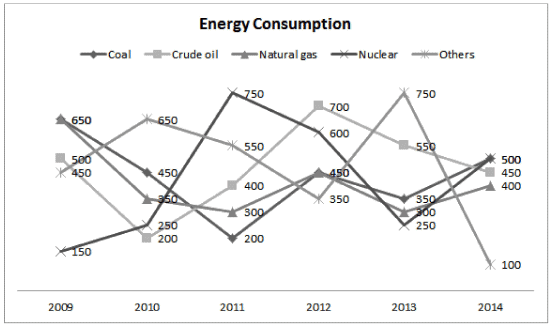Q. Which year registered the highest external trade in the energy sector?

Detailed Solution for IIFT Data Interpretation MCQ - 2 - Question 1

External trade = | Imports coal | + | Imports crude oil I + I Imports Natural gas I + I Imports Nuclear I + I Imports others I
Imports = Consumption - Production
In the equation above, exports have also been considered as imports i.e. a negative value of imports would actually mean an export.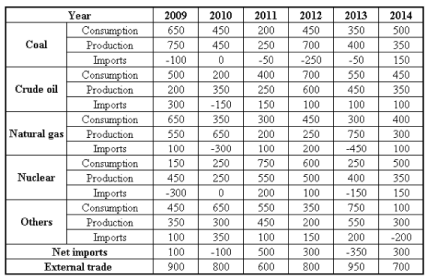From the table, the external trade is highest in the year 2013.

IIFT Data Interpretation MCQ - 2 - Question 2

### Analyze the charts given below that show the energy consumption and energy production of XYZ country from various sources. The difference in production and consumption of energy from any source is bridged through external trade (i.e. export or import) of energy from the same source. All the values are given in Terawatt-hours (Twh).Q. In which year are the net imports of all the categories taken together the lowest?

Detailed Solution for IIFT Data Interpretation MCQ - 2 - Question 2

Consider the solution to the first question.
Among the given options, net imports are lowest in 2009.
Hence, option 1.

IIFT Data Interpretation MCQ - 2 - Question 3

### Analyze the charts given below that show the energy consumption and energy production of XYZ country from various sources. The difference in production and consumption of energy from any source is bridged through external trade (i.e. export or import) of energy from the same source. All the values are given in Terawatt-hours (Twh).Q. Which of the following statement(s) is/are true? I. In each year, net exports are always less than the external trade. II. Coal is always exported. III. Net import of energy from at least one source is nil from 2009-2014.

Detailed Solution for IIFT Data Interpretation MCQ - 2 - Question 3

Net exports = - net imports
From the table, it is always less than the external trade.
Thus, statement I is true.
Import of coal in 2014 is 150TWh.
Thus, statement II is false.
Net import of nuclear energy = -300 + 0 + 200 + 100 -150 + 150 = 0
Thus, statement III is true.

IIFT Data Interpretation MCQ - 2 - Question 4

Group Question
Answer the following question based on the information given below.

Eight friends Raju, Ravi, Ramesh, Rahul, Arjun, Arvind, Anish and Atul are skilled at making Handicraft items. In order to make money using their skills, they decide to make a certain number of handicraft items and sell them at a Handicraft Expo. They decided to minimize time and maximize their productivity by forming two groups. The people whose names start with the letter ‘R’ form Group 1 and the people whose names start with the letter ‘A’ form Group 2. Each person from Group 1 forms a team each person from Group 2 to produce a certain number of handicraft items of a particular type, the details of which are given in the tables below.
Table 1 gives details of the type of handicraft item produced by each team.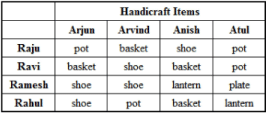Table 2 gives details of the number of handicraft items produced by each team.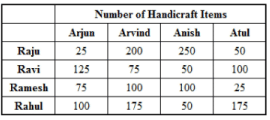Table 3 gives the cost price and selling price of one item of each type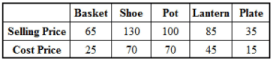The money (Cost Price and Profit) is shared according to the number of items each one has worked on. For example, if Rahul and Arvind made 175 pots, the total cost price and the profit made by selling those pots would be shared equally.

Q. Who has worked on the maximum number of handicraft items?

Detailed Solution for IIFT Data Interpretation MCQ - 2 - Question 4

The number of handicraft items worked on by any person is directly given in table 2.
Raju, Rahul, Arvind and Anish have worked on 525, 500, 550 and 5
450 items respectively.
Thus, among these four people, Arvind has worked on the maximum number of handicraft items.
Hence, option 3.

IIFT Data Interpretation MCQ - 2 - Question 5

Eight friends Raju, Ravi, Ramesh, Rahul, Arjun, Arvind, Anish and Atul are skilled at making Handicraft items. In order to make money using their skills, they decide to make a certain number of handicraft items and sell them at a Handicraft Expo. They decided to minimize time and maximize their productivity by forming two groups. The people whose names start with the letter ‘R’ form Group 1 and the people whose names start with the letter ‘A’ form Group 2. Each person from Group 1 forms a team each person from Group 2 to produce a certain number of handicraft items of a particular type, the details of which are given in the tables below.
Table 1 gives details of the type of handicraft item produced by each team.Table 2 gives details of the number of handicraft items produced by each team.Table 3 gives the cost price and selling price of one item of each typeThe money (Cost Price and Profit) is shared according to the number of items each one has worked on. For example, if Rahul and Arvind made 175 pots, the total cost price and the profit made by selling those pots would be shared equally.

Q. For how many teams was the total selling price more than Rs. 15,000?

Detailed Solution for IIFT Data Interpretation MCQ - 2 - Question 5

The total selling prices for the teams are given in the table below: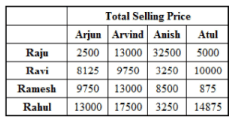The total selling price was more than Rs. 15,000 for 2 teams: Raju-Anish and Rahul-Arvind.

IIFT Data Interpretation MCQ - 2 - Question 6

Eight friends Raju, Ravi, Ramesh, Rahul, Arjun, Arvind, Anish and Atul are skilled at making Handicraft items. In order to make money using their skills, they decide to make a certain number of handicraft items and sell them at a Handicraft Expo. They decided to minimize time and maximize their productivity by forming two groups. The people whose names start with the letter ‘R’ form Group 1 and the people whose names start with the letter ‘A’ form Group 2. Each person from Group 1 forms a team each person from Group 2 to produce a certain number of handicraft items of a particular type, the details of which are given in the tables below.
Table 1 gives details of the type of handicraft item produced by each team.Table 2 gives details of the number of handicraft items produced by each team.Table 3 gives the cost price and selling price of one item of each typeThe money (Cost Price and Profit) is shared according to the number of items each one has worked on. For example, if Rahul and Arvind made 175 pots, the total cost price and the profit made by selling those pots would be shared equally.

Q. For how many teams was the ratio of the total cost price to the total selling price less than 0.5?

Detailed Solution for IIFT Data Interpretation MCQ - 2 - Question 6

The ratio of cost price to selling price is less than 0.5 for only baskets and plates.
Hence, any team that has made baskets or plates satisfies the given conditions. 4 teams have made baskets and 1 team has made plates i.e. 5 teams satisfy the condition.
Hence, option 3.

IIFT Data Interpretation MCQ - 2 - Question 7

Eight friends Raju, Ravi, Ramesh, Rahul, Arjun, Arvind, Anish and Atul are skilled at making Handicraft items. In order to make money using their skills, they decide to make a certain number of handicraft items and sell them at a Handicraft Expo. They decided to minimize time and maximize their productivity by forming two groups. The people whose names start with the letter ‘R’ form Group 1 and the people whose names start with the letter ‘A’ form Group 2. Each person from Group 1 forms a team each person from Group 2 to produce a certain number of handicraft items of a particular type, the details of which are given in the tables below.
Table 1 gives details of the type of handicraft item produced by each team.Table 2 gives details of the number of handicraft items produced by each team.Table 3 gives the cost price and selling price of one item of each typeThe money (Cost Price and Profit) is shared according to the number of items each one has worked on. For example, if Rahul and Arvind made 175 pots, the total cost price and the profit made by selling those pots would be shared equally.

Q. Which of the following statement(s) is/are true?
I. The total profit earned by Atul is Rs. 6,000
II. The total selling price of all the items that Ramesh has worked on is Rs. 32,125
III. The team of Raju and Arjun had the lowest total selling price of the items they had produced.

Detailed Solution for IIFT Data Interpretation MCQ - 2 - Question 7

Statement III can be directly verified from the solutions to one of the earlier questions.
The team of Ramesh-Atul has the lowest selling price.
Hence, statement III is false.
Hence, options 2 and 4 can be eliminated.
Since II is present in both the remaining options, you can directly assume it to be true.
Hence, just verify I.
The total profit earned by Atul = (50/2) * 30 + (100/2) x 30 + (25/2 x 20) + (175/2 x 40) = Rs. 6,000
Thus, statement I is true.
Hence, option 1.

IIFT Data Interpretation MCQ - 2 - Question 8

Eight friends Raju, Ravi, Ramesh, Rahul, Arjun, Arvind, Anish and Atul are skilled at making Handicraft items. In order to make money using their skills, they decide to make a certain number of handicraft items and sell them at a Handicraft Expo. They decided to minimize time and maximize their productivity by forming two groups. The people whose names start with the letter ‘R’ form Group 1 and the people whose names start with the letter ‘A’ form Group 2. Each person from Group 1 forms a team each person from Group 2 to produce a certain number of handicraft items of a particular type, the details of which are given in the tables below.
Table 1 gives details of the type of handicraft item produced by each team.Table 2 gives details of the number of handicraft items produced by each team.Table 3 gives the cost price and selling price of one item of each typeThe money (Cost Price and Profit) is shared according to the number of items each one has worked on. For example, if Rahul and Arvind made 175 pots, the total cost price and the profit made by selling those pots would be shared equally.

Q. For which type of handicraft was the total number of items 1Marks produced the highest?

Detailed Solution for IIFT Data Interpretation MCQ - 2 - Question 8

The total number of items for each type of handicraft is:
Baskets = 125 + 200 + 50 + 50 = 425
Shoes = 75 + 100 + 75 + 100 + 250 = 600
Pots = 25 + 175 + 50 + 100 = 350
Lanterns = 100 + 175 = 275
Hence, option 2.

IIFT Data Interpretation MCQ - 2 - Question 9

Group Question
Answer the following question based on the information given below.

Year-wise break-up of number of educational institutions in India: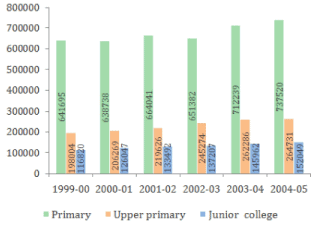Q. Which of the following year has seen the maximum percentage growth of Upper primary schools over the previous year?

Detailed Solution for IIFT Data Interpretation MCQ - 2 - Question 9

Percentage growth of Upper primary schools: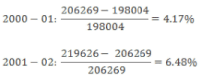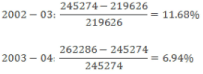The year 2002-03 has seen the maximum growth.
Hence, option 3.

IIFT Data Interpretation MCQ - 2 - Question 10

Year-wise break-up of number of educational institutions in India:Q. Which of the following statements is definitely true?

Detailed Solution for IIFT Data Interpretation MCQ - 2 - Question 10

Option (1):

Percentage share of Upper primary institutions in 1999 - 00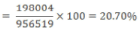Percentage share of Upper primary institutions in 2000 - 01 =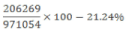Increase = 21.24 - 20.70 = 0.54%
Percentage share of Prim ary institutions in 1999 - 00 =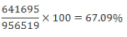Percentage share of Prim ary institutions in 2000 - 01 =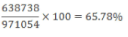Decrease = 67.09 - 65.78 = 1.31%

Since the increase in percentage share of Upper primary institutions in 2000-01 is less than the decrease in percentage share of Primary institutions in the same period, the statement is false.

Option (2):

Percentage share o f Upper prim ary institutions in 2003 - 04 =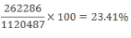Percentage share o f Upper prim ary institutions in 2004 - 05 =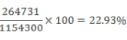Decrease = 23.41 - 22.93 = 0.48%
Percentage share o f Prim ary institutions in 2003 - 04 =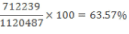Percentage share o f Prim ary institutions in 2004 - 05 =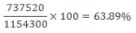Increase = 63.89 - 63.57 = 0.32%

Since the decrease in percentage share of Upper primary institutions in 2004-05 is more than the increase in percentage share of Primary institutions in the same period, the statement is false.

Option (3):

From option (1), we have already seen that the year 2000-01 has also seen a decline in the percentage share of primary institutions, so the statement is false.

Option (4):

Percentage sh are o f Junior colleges in 2002 -03 =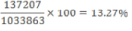Percentage share of Junior colleges in 2003 - 04 =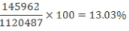Decrease = 13.27 - 13.03 = 0.24%

Percentage share of Primary institutions in 2002 - 03 =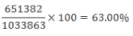Percentage share of Primary institutions in 2003 - 04 =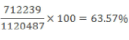Increase = 63.57 - 63.00 = 0.57%

Since the decrease in percentage share of Junior colleges in total in the year 2003-04 over the previous years is less than the increase in percentage share of primary institutions in the same period, the statement is true.

Hence, option 4.

IIFT Data Interpretation MCQ - 2 - Question 11

Year-wise break-up of number of educational institutions in India:Q. Which of the following statement(s) is/are definitely true?

Detailed Solution for IIFT Data Interpretation MCQ - 2 - Question 11

Option (1):

The statement is false as the number of Primary institutions first
decreased in 2000-01, then increased in 2001-02 and again decreased in 2002-03.
Options (2) and (3) are true.
Hence, option 4.

IIFT Data Interpretation MCQ - 2 - Question 12

Year-wise break-up of number of educational institutions in India:Q. What is the number of years in which the total number of Upper primary schools and Junior colleges is less than half of the number of Primary schools?

Detailed Solution for IIFT Data Interpretation MCQ - 2 - Question 12

The total number of Upper primary schools and Junior colleges is less than half the number of Primary schools only in the year 1999- 00.
Hence, option 1.

IIFT Data Interpretation MCQ - 2 - Question 13

Year-wise break-up of number of educational institutions in India:Q. If in the year 2005-06, the number of Upper primary schools grows by the highest percentage growth recorded in the last 6 years (for Upper primary schools), then what is the expected number of Upper primary schools in 2005-06?

Detailed Solution for IIFT Data Interpretation MCQ - 2 - Question 13

In the solution to the 1st problem of this set, it is has been found that the highest percentage growth for Upper primary schools was 11.68% in 2002-03.
Hence, the number of Upper primary schools in 2005-06 = 264731 x 1.1168 = 295651.58*295652
Hence, option 2.

IIFT Data Interpretation MCQ - 2 - Question 14

Group Question
Answer the following question based on the information given below.

The table below shows production area, production quantity, export quantity, import quantity, export and import value of dry fruits over the period of five years in a country.
The production is consumed in two ways - export and domestic consumption.
The export and import values in crores are in the local currency of the country.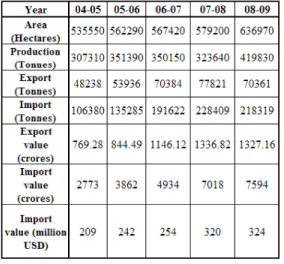Q. Approximately, what percentage of domestic consumption are imports of dry fruits during 2005-2006?

Detailed Solution for IIFT Data Interpretation MCQ - 2 - Question 14

In 2005-2006, the domestic consumption is 351390 - 53936 = 297454 tonnes

Imports in that year = 135285
The imports as a percentage of domestic consumption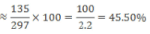Hence, option 2.

IIFT Data Interpretation MCQ - 2 - Question 15

The table below shows production area, production quantity, export quantity, import quantity, export and import value of dry fruits over the period of five years in a country.
The production is consumed in two ways - export and domestic consumption.
The export and import values in crores are in the local currency of the country.Q. What is the value of exports in 2008-09 in millions of US dollars, considering the same foreign exchange value as imports in that year?

Detailed Solution for IIFT Data Interpretation MCQ - 2 - Question 15

As 7594 crores in local currency correspond to 324 million USD, Each unit of local currency in 2008-2009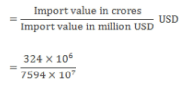The value of exports in the year 2008-2009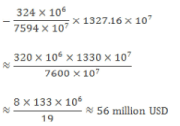The closest answer is option 2.
Hence, option 2.

IIFT Data Interpretation MCQ - 2 - Question 16

The table below shows production area, production quantity, export quantity, import quantity, export and import value of dry fruits over the period of five years in a country.
The production is consumed in two ways - export and domestic consumption.
The export and import values in crores are in the local currency of the country.Q. Which of the following statements is true?

Detailed Solution for IIFT Data Interpretation MCQ - 2 - Question 16

Consider option 1:
Production per hectare in 2004-2005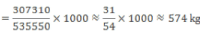The statement in option 1 is false.

Consider option 2:

Production per hectare in 2008-2009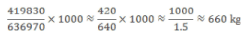The statement in option 2 is false.

Consider option 3: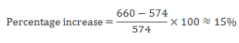The statement in option 3 is true.

Hence, option 3.

IIFT Data Interpretation MCQ - 2 - Question 17

The table below shows production area, production quantity, export quantity, import quantity, export and import value of dry fruits over the period of five years in a country.
The production is consumed in two ways - export and domestic consumption.
The export and import values in crores are in the local currency of the country.Q. Which of the following statements is true?

Detailed Solution for IIFT Data Interpretation MCQ - 2 - Question 17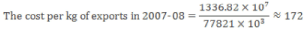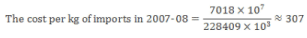The difference in costs of exports and imports = 307 - 172 = Rs. 135

Hence, option 1.

IIFT Data Interpretation MCQ - 2 - Question 18

The table below shows production area, production quantity, export quantity, import quantity, export and import value of dry fruits over the period of five years in a country.
The production is consumed in two ways - export and domestic consumption.
The export and import values in crores are in the local currency of the country.Q. Which of the following statements is false?

Detailed Solution for IIFT Data Interpretation MCQ - 2 - Question 18

Consider option 1:
Total number of hectares required in 2013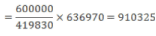Additional area needed = 910325 - 636970 = 273355 hectares or 2.73 lakh hectares.

Consider option 2:

Total number of hectares required in 2013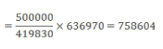Additional area needed = 758604 - 636970 = 121634 hectares or 1.22 lakh hectares.

Consider option 3:

Total number of hectares required in 2013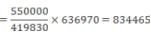Additional area needed = 834465 - 636970 = 197495 hectares or 1.98 lakh hectares.

Hence, option 3.

## IIFT Mock Test Series

1 docs|46 tests
 Use Code STAYHOME200 and get INR 200 additional OFF Use Coupon Code
Information about IIFT Data Interpretation MCQ - 2 Page
In this test you can find the Exam questions for IIFT Data Interpretation MCQ - 2 solved & explained in the simplest way possible. Besides giving Questions and answers for IIFT Data Interpretation MCQ - 2, EduRev gives you an ample number of Online tests for practice

## IIFT Mock Test Series

1 docs|46 tests

### How to Prepare for CAT

Read our guide to prepare for CAT which is created by Toppers & the best Teachers